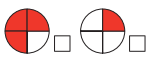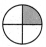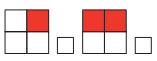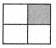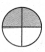Tamilnadu State Board New Syllabus Samacheer Kalvi 4th Maths Guide Pdf Term 2 Chapter 6 Fraction Ex 6.6 Textbook Questions and Answers, Notes.

## Tamilnadu Samacheer Kalvi 4th Maths Guide Term 2 Chapter 6 Fraction Ex 6.6

I. Circle the greator fractions

Question 1.
$$\frac{1}{3}, \frac{2}{3}$$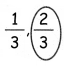Question 2.
$$\frac{3}{4}, \frac{1}{4}$$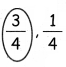Question 3.
$$\frac{2}{5}, \frac{4}{5}$$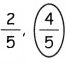Question 4.
$$\frac{6}{8}, \frac{3}{8}$$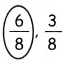Question 5.
$$\frac{4}{10}, \frac{3}{10}$$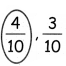Question 6.
$$\frac{2}{9}, \frac{7}{9}$$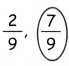\II. Tick the small fractions

Question 1.